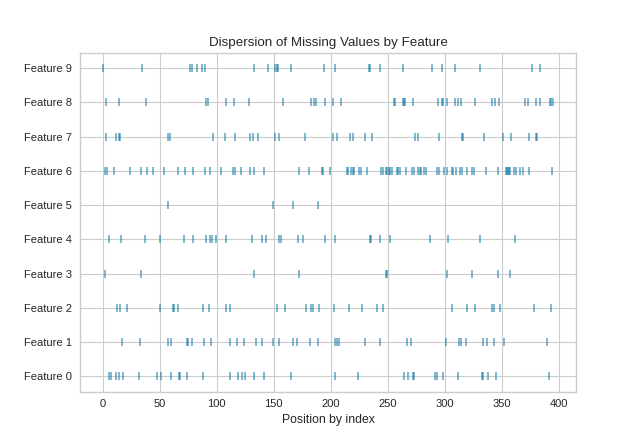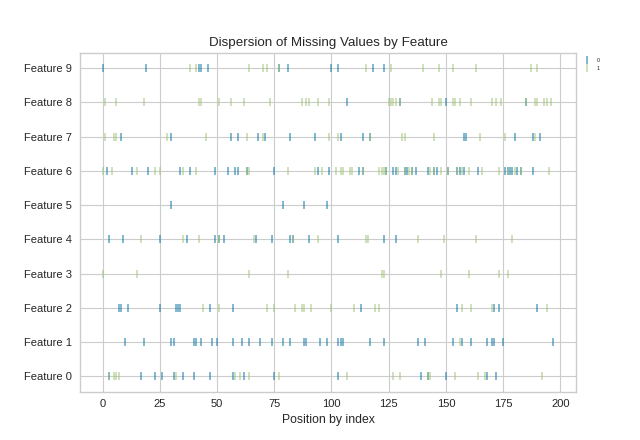# MissingValues Dispersion¶

The MissingValues Dispersion visualizer creates a chart that maps the position of missing values by the order of the index.

## Without Targets Supplied¶

import numpy as np

from sklearn.datasets import make_classification
from yellowbrick.contrib.missing import MissingValuesDispersion

X, y = make_classification(
n_samples=400, n_features=10, n_informative=2, n_redundant=3,
n_classes=2, n_clusters_per_class=2, random_state=854
)

# assign some NaN values
X[X > 1.5] = np.nan
features = ["Feature {}".format(str(n)) for n in range(10)]

visualizer = MissingValuesDispersion(features=features)

visualizer.fit(X)
visualizer.show()## With Targets (y) Supplied¶

import numpy as np

from sklearn.datasets import make_classification
from yellowbrick.contrib.missing import MissingValuesDispersion

X, y = make_classification(
n_samples=400, n_features=10, n_informative=2, n_redundant=3,
n_classes=2, n_clusters_per_class=2, random_state=854
)

# assign some NaN values
X[X > 1.5] = np.nan
features = ["Feature {}".format(str(n)) for n in range(10)]

# Instantiate the visualizer
visualizer = MissingValuesDispersion(features=features)

visualizer.fit(X, y=y) # supply the targets via y
visualizer.show()## API Reference¶

Dispersion visualizer for locations of missing values by column against index position.

class yellowbrick.contrib.missing.dispersion.MissingValuesDispersion(alpha=0.5, marker='|', classes=None, **kwargs)[source]

Bases: yellowbrick.contrib.missing.base.MissingDataVisualizer

The Missing Values Dispersion visualizer shows the locations of missing (nan) values in the feature dataset by the order of the index.

When y targets are supplied to fit, the output dispersion plot is color coded according to the target y that the element refers to.

Parameters
alphafloat, default: 0.5

A value for bending elments with the background.

markermatplotlib marker, default: |

The marker used for each element coordinate in the plot

classeslist, default: None

A list of class names for the legend. If classes is None and a y value is passed to fit then the classes are selected from the target vector.

kwargsdict

Keyword arguments that are passed to the base class and may influence the visualization as defined in other Visualizers.

Examples

>>> from yellowbrick.contrib.missing import MissingValuesDispersion
>>> visualizer = MissingValuesDispersion()
>>> visualizer.fit(X, y=y)
>>> visualizer.show()

Attributes
features_np.array

The feature labels ranked according to their importance

classes_np.array

The class labels for each of the target values

draw(self, X, y, **kwargs)[source]

Called from the fit method, this method creates a scatter plot that draws each instance as a class or target colored point, whose location is determined by the feature data set.

If y is not None, then it draws a scatter plot where each class is in a different color.

draw_multi_dispersion_chart(self, nan_locs)[source]

Draws a multi dimensional dispersion chart, each color corresponds to a different target variable.

finalize(self, **kwargs)[source]

Sets the title and x-axis label and adds a legend. Also ensures that the y tick labels are set to the feature names.

Parameters
kwargs: generic keyword arguments.

Notes

Generally this method is called from show and not directly by the user.

get_nan_locs(self, **kwargs)[source]

Gets the locations of nans in feature data and returns the coordinates in the matrix